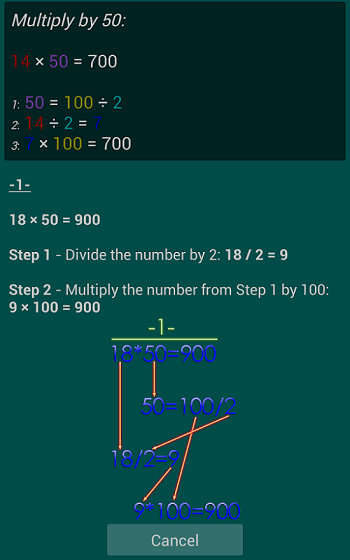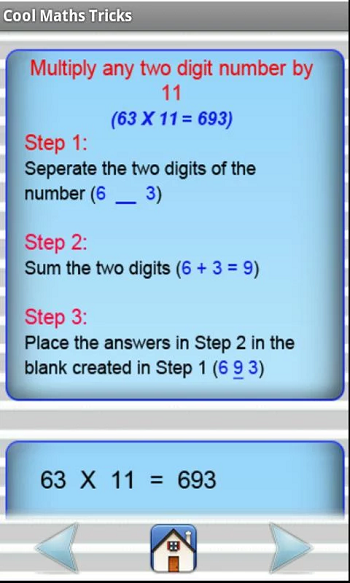These days, smartphones serve several purposes and one such use is education. There are several applications that are aimed at rendering educational services. In this category in the Play Store, there are numerous mathematical applications that will provide different tricks to the students to go about with the subject easily. Here we have listed some of the five math trick applications for the Android devices.

Math Tricks

Math Tricks app is designed for those students who want to learn exciting mathematical tricks to fasten up the calculating process. These mathematical tricks will help in solving a part of the arithmetical problems at a faster pace than usual. It will also help the students to sharpen their basics such as the multiplication table. Learning these mathematical tricks will help users to show off their talent in mathematics. Use these skills to ensure quick calculation skills that will help in saving a lot of precious time.Fun Math Tricks Lite

With the Fun Math Tricks Lite application, users can learn the tricks related to mathematics and astonish their friends, colleagues and relatives. The application lets users to use their mental math tricks to solve tough math problems. This can be done by working out based on the tricks with widget. This app is very useful for the candidates to appear in competitive tests such as SAT, PSAT, GRE, GMAT, ACT, MCAT.Rapid Math Tricks & Tips

Rapid Math Tricks & Tips application demonstrates several time-saving tips and tricks that will help in performing common math calculations. There are samples displayed to demonstrate each trick in a step-by-step manner. Users can amaze their friends with their incredible calculations that were considered difficult to master. It details how to master difficult problems in addition, subtraction, multiplication, and division quickly and easily without using a calculator.Mathemagic

Users can be a magician in front of their friends by letting them think of a number and predicting it using the app. Mathemagic is a math based mind reading application that is based on math tricks such as algebra. This app will let users to guess the age, date of birth, cards chosen, numbers that are chosen and read others mind. It uses old math tricks to accomplish the same. Users can enjoy the magic involved in math using this application.Cool Maths Tricks

Cool Maths Tricks helps users in carrying out multiplication, division, subtraction and squaring at lightning fast speeds. Users can learn and practice the mathematical tricks involved in Vedic math calculations in an engaging way using this app. Users can learn the math tricks and practice them by means of quizzes. The highlight of this app is that it helps in carrying out big problems easily and train them in competitive exams like GMAT, SAT, PSAT, GRE, CAT, ACT, MCAT.Conclusion

These are only a handful of applications that are available in the Play Store to learn math tricks. There are many others in the category and you can try them on your smartphone to enhance your skills.

This site uses Akismet to reduce spam. Learn how your comment data is processed.